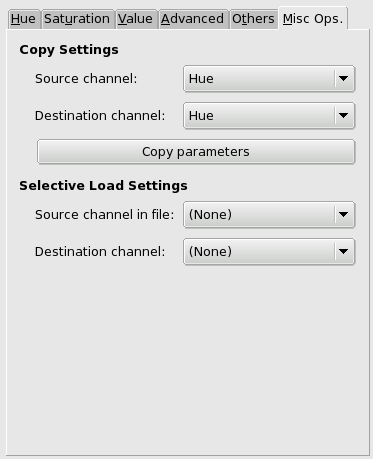13.8. CML Explorer

13.8.1. Overview

Figure 16.293. Example for the CML Explorer filter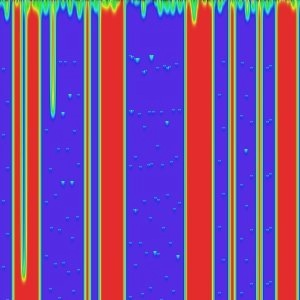Filter CML Explorer applied with default options

This filter is the king of texture creating filters. It is extremely efficient but very complex. It uses a mathematical method named Cellular Automata [WKPD-CA].

13.8.2. Activating the filter

You can find this filter from the image menu through FiltersRenderPatternCML Explorer.

13.8.3. Options

General Options

Filter options are distributed among Hue,Saturation, Value, Advanced, others and Misc.ops tabs. Some more options are available. They will be described in following section.

Preview

This filter offers you a Preview where you can see the result of your settings before they are applied to the image.

New seed; Fix seed; Random seed

Random plays a large part in creating patterns. With these options, you can influence the way random is generated. By clicking on the button, you can force random to use a new source of random. The preview will show you the result. lets you keep the same seed and so to reproduce the same effect with the filter. generates a random seed at random.

Open; Save

With these both command buttons you can save pattern settings in a file, and to get them back later.

“CML Explorer” filter options(Hue)

Figure 16.294. Hue tab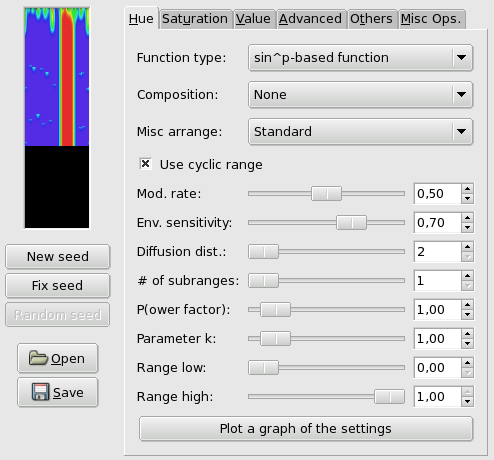This filter works in the HSV color model. In this tab, you can set options for Hue.

Function type

In this drop-down list, you can select the method that will be used to treat the current layer. These methods are:

• Keep image's values: With this option, image hue values will be kept.

• Keep the first value: With this option, starting color will be standard cyan.

• Fill with k parameter, k{x(1-x)}^p, k{x(1-x)}^p stepped, kx^p, kx^p stepped, k(1-x^p), k(1-x^p) stepped: pattern look will depend on k that you will set later in options.

• Delta function, Delta function stepped: FIXME

• sin^p based Function, sin^p stepped: These options create wave-like patterns, like aurora borealis or curtain folds.

Composition

Here, these options concern Hue. You can select:

None, Max(x, -), Max(x+d, -), Max(x-d, -), Min(x, -), Min(x+d, -), Min(x-d, -), Max(x+d, -), (x < 0,5), Max(x+d, -), (0,5 < x), Max(x-d, -), (x < 0,5), Max(x-d, -), (0,5 < x), Min(x+d, -), (x < 0,5), Min(x+d, -), (0,5 < x), Min(x-d, -), (x < 0,5) and Min(x-d, -), (0,5 < x).

A book could be filled with results of all these functions. Please, experiment!

Misc. Arrange

This drop-down list offers you some other parameters:

Standard, Use average value, Use reverse value, With random power (0,10), With random power (0,1), with gradient power (0,1), Multiply rand. value (0,1), Multiply rand. value (0,2), Multiply gradient (0,1) and With p and random (0,1).

Also a book would be necessary to explain all possibilities of these parameters.

Use cyclic range

//TODO

Mod. rate

With this slider and the input box, you can set modification rate from 0.0 to 1.0. Low value results in a lined pattern.

Env. sensitivity

Value is from 0.0 to 1.0

Diffusion dist.

Diffusion distance: from 2 to 10.

# of subranges

Number of sub-rangers: from 1 to 10.

(P)ower factor

With this option you can influence the Function types using the p parameter. Value from 0.0 to 10.0.

k Parameter

With this option you can influence the Function types using the k parameter. Value from 0.0 to 10.0.

Range low

Set lower limit of hue that will be used for calculation. values vary from 0.0 to 1.0.

Range high

Set the upper limit of hue that will be used for calculation. Variations are from 0.0 to 1.0.

Plot a graph of the settings

By clicking on this large button, you can open a window that displays the graph of hue present settings.

Figure 16.295. Function graph of present settings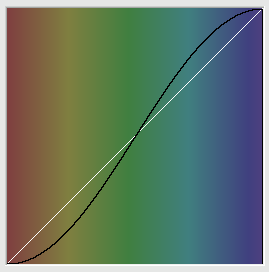“CML Explorer” filter options(Saturation)

Figure 16.296. Saturation tab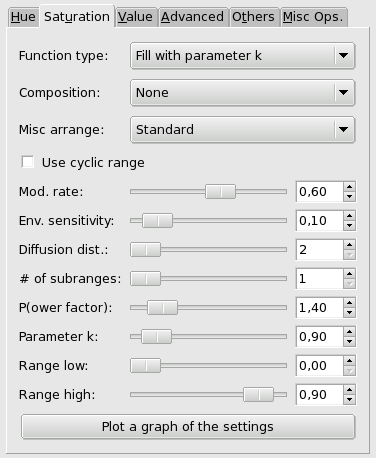In this tab, you can set how Saturation component of the HSV color model will be used in pattern calculation.

These options are similar to Hue tab options.

“CML Explorer” filter options (Value)

Figure 16.297. Value tab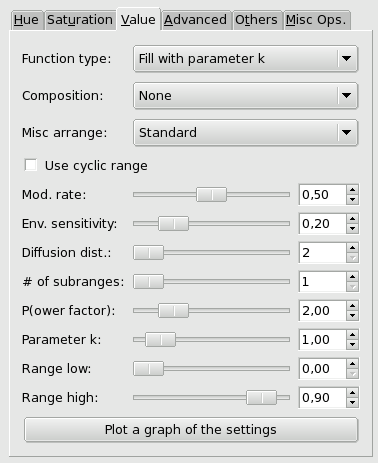In this tab, you can set how the Value (Luminosity) component of the HSV color model will be used in pattern calculation.

These options are similar to Hue tab options.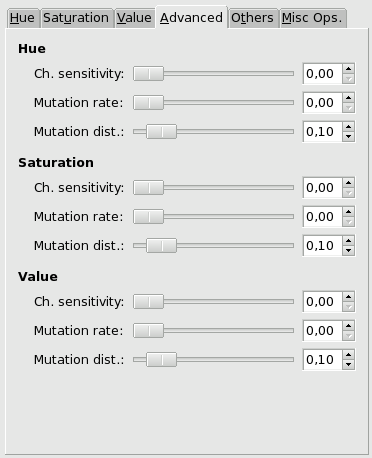These tab settings apply to the three HSV channels.

Channel Sensitivity

// TODO

Mutation rate

// TODO

Mutation distance

//TODO

“CML Explorer” filter options (Others)

Figure 16.299. Others tab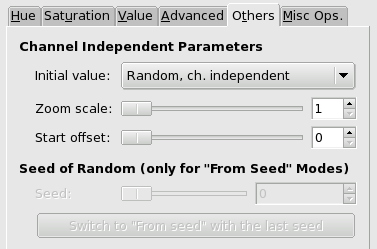In this tab, you can find various parameters about image display and random intervention.

Initial value

// TODO

Zoom factor

// TODO

Start offset

// TODO

Seed of random

// TODO

“CML Explorer” filter options (Misc.ops)

Figure 16.300. Miscellaneous options tab